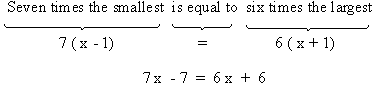Home MonkeyNotes Printable Notes Digital Library Study Guides Study Smart Parents Tips College Planning Test Prep Fun Zone Help / FAQ How to Cite New Title Request

 Example A number exceeds another number by 12. If the sum of two number is 30 find the smaller number. Solution : We are looking for the "smaller number" Let the small number be ' x ' Therefore the larger number is x + 12 Setting up the equation as : If the sum of two numbers is x + ( x + 12) = 30 \ 2 x + 12 = 30 \ 2 x = 30 - 12 . . . (on transposing ) \ x = 18 Therefore, the smaller number is 17. Example Three consecutive natural numbers are such that seven times of the smallest is equal to six times the largest. Find the numbers. Solution : You are looking for three consecutive natural numbers. Let them be x -1, x, x+1 Therefore the smallest is x-1 and the largest is x +1 Setting up the equation as -7 x - 6 x = 6 + 7 . . . ( on transposing ) x = 13 \ x - 1 = 13 -1 x - 1 = 12 and x +1 = 13 + 1 = 14 i.e. The three consecutive natural numbers are 12, 13, 14. Index 9.1 - Definition and Solving Techniques9.2 - Use of Simultaneous Linear Equations Chapter 1
All Contents Copyright © All rights reserved.
Further Distribution Is Strictly Prohibited.

 Search: All Products Books Popular Music Classical Music Video DVD Toys & Games Electronics Software Tools & Hardware Outdoor Living Kitchen & Housewares Camera & Photo Cell Phones Keywords: